Resultant of coplanar force system is the summation of all forces acting on the rigid body on the same plane. It produces the same effect that each single force can produce on the body.

Resultant forces of a system can be determined by using two methods:

• Graphical Method
• Analytical Method

Determine the Resultant of Collinear Coplanar Forces

We know that collinear coplanar forces have common line of action in the same plane. First we will discuss the analytical method of determining the resultant of collinear coplanar forces.

1stAnalytical Method:

Let us consider a system of three forces F1, F2 and F3act on the rigid body as shown in Fig. 1.36 and Fig. 1.37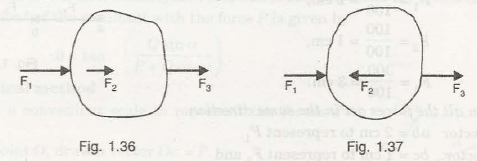It shows all three forces act in the same direction on the rigid body.

It shows two forces act in the same direction and one force acts in opposite direction on the rigid body.

In this condition, for calculating the resultant of coplanar forces, R will be given by-

R = F1 + F2+ F3                                      (For Fig. 1.36)

And,

R = F1– F2 + F3                                      (For Fig. 1.37)

2ndGraphical Method:

While calculating the resultant of coplanar forces, the given number of forces is drawn on a suitable scale as shown in Fig. 1.38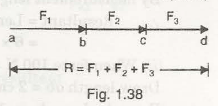Let us consider all forces in the same direction

First force acting on the body be F1 = ab

Second force acting on the body be F2 = bc

Third force acting on the body be F3 = cd

Then,

Resultant force can be given by,

R = F1 + F2 + F3

Or,

R = ab + bc + cd

Suppose two forces act in the same direction and third one is in opposite direction as shown in Fig. 1.39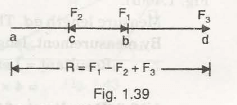First force acting on the body be F1 = ab

Second force acting on the body be F2 = – bc

Third force acting on the body be F3 = cd

Then,

Resultant force can be given by,

R = F1 – F2 + F3

Or,

R = ab – bc + cd

For example:

Determine the resultant of forces when three collinear forces of 100 N, 200N and 300 N act on the rigid body.

1. When all forces in same direction
2. When force 100N acts in opposite direction

Solution:

Given Data:

F1 = 100N

F2= 200N

F3 = 300N

A. When all forces in same direction

The resultant can be given by-

From Analytical Method,

R = F1 + F2 + F3

= 100 + 200 + 300

= 600N

B. When force 100N acts in opposite direction

The resultant can be given by-

From Analytical Method,

R = – F1 + F2 + F3

= – 100 + 200 + 300

= 400N

Determine the Resultant of Concurrent Coplanar Forces

We know that concurrent coplanar forces meet at a single point in the same plane. To determine the resultant of collinear coplanar forces, we consider two cases-

1. Two forces act at single point

Analytical Method:

Let us consider a system of two forcesP and Qact at a single pointO as shown in Fig. 1.41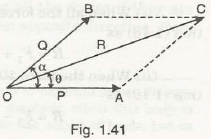Angle between these two forces be

We know that,

According to the Parallelogram Law of Forces, the diagonal of two forces gives the value of resultant R. If the angle between the resultant force R and horizontal line be , then

Magnitude of resultant can be given by,

R = √(P2 + Q2 + 2PQ cos ∝)

This is also called as the cosine law method.

For determining the direction of resultant, we can write the equation as-

tan θ = (Q sin ∝)/( P + Q cos ∝)

Or,

θ = tan–1((Q sin ∝)/( P + Q cos ∝))

Graphical Method:

Let us draw two forces P and Q from point O taking suitable scales

Draw vector Oa for force P

Draw vector Ob for force Q as shown in Fig. 1.42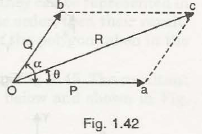Complete the parallelogram by drawing dotted parallel lines to Oa and Ob

Join Oc- It shows the diagonal of the parallelogram

Oc represents the resultant of forces, R

Mark ⦟bOa as∝ and Mark ⦟cOa as θ

Now,

Consider another step to determine the resultant of forces as shown in Fig. 1.43,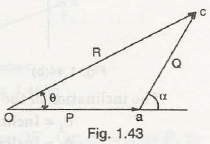Draw a vector line Oa similar in scale as compare to the previous and parallel to it

Draw another vector line at an angle ∝from point a equal to ac

Join Oc

So, line Oa shows force P and ac shows force Q

The line joining O and c shows the resultant of two forces

Angle between the resultant and horizontal force θ gives its direction

1. Two and more forces act at single point

Analytical Method:

For determining the resultant of two or more forces, we consider the rectangular components methods. We convert all the forces into its horizontal and vertical components for algebraic summation.

Let us consider four forces F1, F2, F3 and F4act on a body at a point O as shown in the Fig. 1.44-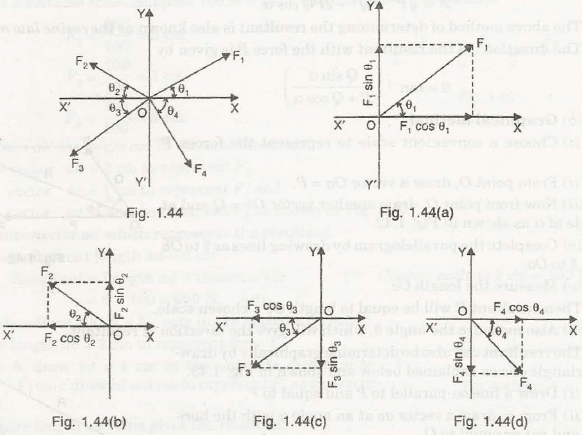Consider Fig. 1.44 (a), we can see how force F1 is solved in its horizontal component and vertical component as-

Angle made by force F1 with the horizontal axis be θ1

Then,

Horizontal Component = F1 cos θ1

Vertical Component = F1sinθ1

Similarly,

Consider Fig. 1.44 (b), Fig. 1.44 (c) and Fig. 1.44 (d), the horizontal components and vertical componentscan be given by-

Angle made by force F2 with the horizontal axis be θ2

Angle made by force F3 with the horizontal axis be θ3

Angle made by force F3 with the horizontal axis be θ3

Then,

Horizontal Component = F2 cos θ2, F3 cos θ3 and F4 cos θ4

Vertical Component = F2 sin θ2, F3 sin θ3 and F4 sin θ4

Form the rectangular components method, algebraic summation of components will be given by-

For Horizontal Components,

∑▒〖H=〗F1 cos θ1– F2 cos θ2 – F3 cos θ3¬¬+ F4 cos θ4

And,

∑▒〖V=〗F1 sin θ1+ F2 sin θ2 – F3 sin θ3– F4 sin θ4

For determining the direction of resultant forces,

tan θ = (∑▒H)/(∑▒V)

Or,

θ = tan–1((∑▒H)/(∑▒V))

Graphical Method:

While determining the resultant of coplanar concurrent force system, we consider the polygon law of forces.

Suppose four forces F1, F2, F3 and F4act on a body at a point O as shown in the Fig. 1.45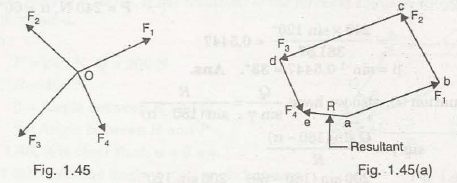If these coplanar forces represent its magnitude and direction, then the resultant can be determined by considering the closing side of the polygon in same order as shown in Fig. 1.45 (a),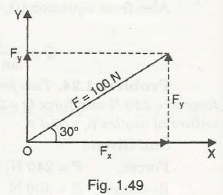Draw vector ab parallel to force F1 on suitable scale

Draw vector bc parallel to force F2

Draw vector cd parallel to force F3

Draw vector de parallel to force F4

Now,

The closing side of the polygon will be given by the line joining point e and a. It is represented by R.

For Example:

Determine the components of force 100 N when acts at an angle of 300 with horizontal as shown in Fig.1.49,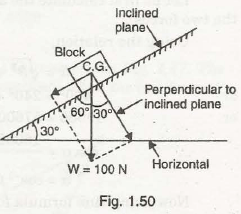Solution:

Given Data:

Force, F = 100 N

Angle, θ = 300

Components of force, F are-

Horizontal Component = F cos θ

= 100 cos 300

= 86.6 N

Vertical Component     = F sinθ

= 100 sin 300

= 50 N

Find the components of weight 100 N which is places on inclined plane as shown in Fig. 1.50. (Consider the inclination angle as 300)Solution:

Given Data:

Weight of the component, W = 100 N

As the weight of the body will act in downward direction the two weight components can be given by-

• Perpendicular component to the inclined plate:

W cos θ= 100 cos 300

= 86.6 N

• Parallel component to the inclined plate:

F sin θ    = 100 sin 300

= 50 N

Resultant of Parallel Coplanar Forces:

If different forces have parallel line of action on a plane, then these are considered as the parallel coplanar forces. These forces are of two types-

1. Like Parallel Force

When two or more forces act on the plane in the same direction, it is called like parallel forces as shown in Fig. 1.58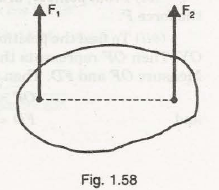It shows two parallel forces F1 and F2 act in the same direction on the rigid body.

1. Unlike Parallel Force

When two or more forces act on the plane in the opposite direction, it is called unlike parallel forces as shown in Fig. 1.59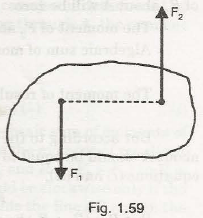It shows two parallel forces F1 and F2 act in opposite direction on the rigid body.

Note:

• If the two parallel forces F1 and F2 actin opposite direction have equal magnitude, it is called unlike equal parallel forces.
• If the two parallel forces F1 and F2 actin opposite direction have unequal magnitude, it is called unlike unequal parallel forces.

For determining the result of two parallel forces, three conditions can be seen-

1. Resultant of two like parallel forces

Let us consider two like parallel forces F1 and F2 act in same direction from point A and point B respectively as shown in Fig. 1.60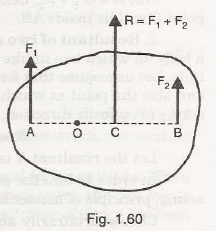To determine its resultant R at point C, we use Varignon’s principle for both the forces.

Taking moments of forces-

Moment of force F1in clockwise direction = F1 x AO              (About point O) —————(1)

Moment of force F2 in anticlockwise direction = F2 x BO      (About point O) —————(2)

Moment of resultant R about point O = R x CO                                             —————-(3)

According to the principle of moments, we can write

Moment of resultant R = Moment of force F1 + Moment of force F2

Substituting the value from equation (1), (2) and (3), we get-

R x CO = – F1 x AO + F2 x BO

We know that, R = F1 + F2

So,

(F1 + F2) x CO = – F1 x AO + F2 x BO

F1 (CO + AO) = F2 (BO – CO)

Or,

F1 (AC) = F2 (BC)

Or,

F_1/F_2  = BC/AC

It shows that distance AB has divided into the ratio of forces F1 and F2and suggest that point C resultant point) lies on the same plane.

Now,

For determining the direction of these two forces, we consider-

Moment of force F1 = 0                                                          (About point A) —————(4)

Moment of force F2 in anticlockwise direction = F2 x AB       (About point A) —————(5)

Moment of resultant R about point A = R x AC                                              —————-(6)

According to the principle of moments,

R x AC = 0 + F2 x AB

We know that, R = F1 + F2

So,

(F1 + F2) x AC = F2 x AB

It suggests that value of AC can be calculated and its value is quite less than value of AB thus it lies somewhere in the line AB.

1. Resultant of two unlike parallel forces which have unequal magnitudes:

Let us consider two unlike parallel forces F1 and F2 act in opposite direction and have unequal magnitude as shown in the figure below-To determine resultant R, we can write for both the forces as-

R = F1– F2

Taking moments of forces-

Moment of force F1 in clockwise direction = F1 x AO             (About point O) —————(1)

Moment of force F2 in clockwise direction = F2 x BO             (About point O) —————(2)

Moment of resultant R about point O = R x CO                                             —————-(3)

So,

We can write-

Moment of resultant R = Moment of force F1 + Moment of force F2

(F1 – F2) x CO = – F1 x AO – F2 x BO

F1 (CO + AO) = F2 (BO – CO)

Or,

F1 (AC) = F2 (BC)

Or,

F_1/F_2  = BC/AC

Since force F1 is greater than force F2, value of BC will be greater than AC. It suggests that its resultant lies on the line joining in the same plane.

Now,

For determining the direction of two forces, we consider-

Moment of force F2 in anticlockwise direction = F2 x AB                   (About point A)

Moment of resultant R about point A = R x AC

According to the principle of moments,

R x AC = 0 + F2 x AB

We know that, R = F1 + F2

So,

(F1– F2) x AC = F2 x AB

It suggests that value of AC can be calculated using the above equation.

1. Resultant of two unlike parallel forces (equal in magnitude):

When two parallel forces equal in magnitude act in opposite direction on a single plane, it forms a couple that rotates the body.

Let us consider one of the forces at point A be F

Perpendicular distance of the arm from the point A be a

Then,

Moment of the couple can be given by-

M = F x a

Note:

Arm of the couple means the perpendicular distance between the forces on the same plane.

For example:

Find the resultant of three parallel forces 100 N, 200 N and 300 N acts on the same direction on a straight line ABC. Also, determine the magnitude of its direction.

Solution:

Given Data:

Distance between the forces are-

AB = 30 cm

BC = 40 cm

AC = 70 cm

Force, F1 at point A = 100 N

Force, F2 at point B = 200 N

Force, F3 at point C = 300 N

So,

Resultant of the above forces can be given by-

R = 100 + 200 + 300

= 600 N

Now,

Taking moment of forces about point A,

Moment of force, F1 about point A = 0

Moment of force, F2 about point A = 200 x 30 = 6000 N

Moment of force, F3 about point A = 300 x 70 = 21000 N

Moment of resultant, R about point A = 600 x

According to the algebraic sum of moments of forces about A, we can write-

Moment of resultant = Moment of force, F1 + Moment of force, F2+ Moment of force, F3

600 x   = 0 + 6000 + 21000

= 27000/ 600

Hence,

= 45 cm

Links of Next Mechanical Engineering Topics:-### Customer Reviews

My Homework Help
Rated 5.0 out of 5 based on 510 customer reviews at